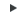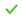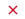# Interpolate Nodes

Create evenly spaced or biased nodes by interpolating between existing nodes in space, on a surface, or on a curved or straight line.

1. From the Geometry ribbon, Nodes/Points tool group, click the Create Points/Nodes tool.
2. From the Geometry ribbon, click the arrow next to the Create tool set, then select Create Points.
3. From the drop-down menu on the guide bar, select Interpolate Nodes.
4. From the second drop-down menu on the guide bar, select from the following:
Option Description
Nodes
1. Select at least two nodes to interpolate nodes between.
Note: Input nodes are specified as a node list, with each node considered as a pair with the next node in the list.
2. In the Number of nodes field, enter the number of nodes to create on a straight line between each selected node pair.
3. Select a bias style: linear, exponential, or bell curve.
4. In the Bias intensity field, enter a value.
Note: Nodes are created in 3D space, and not merely on geometry.
Lines
1. Select lines and at least two nodes to interpolate nodes between.
2. Use the Node List selector to select existing nodes.
Input nodes are specified as a node list, with each node considered as a pair with the next node in the list.
Note: Input nodes are not required to lie on the selected line, as all input nodes are first projected onto the line.
3. In the Number of nodes field, enter the number of nodes to create between each selected node pair.
4. Select a Bias style: linear, exponential, or bell curve.
5. In the Bias intensity field, enter a value.
Locations
1. Select at least two locations to interpolate nodes between.
2. In the Number of nodes field, enter the number of nodes to create between each selected node pair.
3. Select a Bias style: linear, exponential, or bell curve.
4. In the Bias intensity field, enter a value.
Surfaces
1. Select surfaces and at least two nodes to interpolate nodes between.
2. Use the Node List selector to select existing nodes.
Input nodes are specified as a node list, with each node considered as a pair with the next node in the list.
Note: The nodes are not required to lie on the selected surface, as all input nodes are first projected onto the surface.
3. In the Number of nodes field, enter the number of nodes to create between each selected node pair.
4. Select a Bias style: linear, exponential, or bell curve.
5. In the Bias intensity field, enter a value.
5. When complete, from the guide bar, click one of the following:
•- Apply and stay in the tool.
•- Apply and close the tool.
•- Exit the tool without applying.

The following figures illustrate the effect of adding five nodes between the end nodes of a line using the different bias styles with a bias intensity of 5.0.

Nodes are created in 3D space, and not merely on geometry as shown in the previous images.
The following figure illustrates nodes interpolated along a curved line. Five nodes created between two input nodes along a curved line, using linear biasing.
The following figure illustrates nodes interpolated on a surface. The highlighted opposing corner nodes have seven interpolated nodes created between them, using Bellcurve biasing.